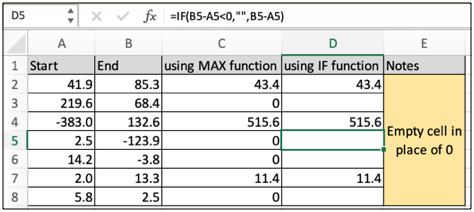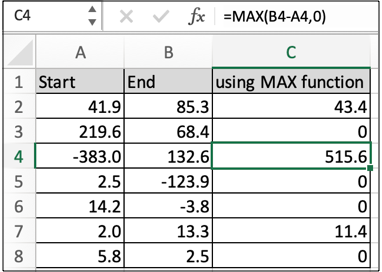# How to eliminate negative values in ExcelIn this article, we will learn How to eliminate negative values in Excel.
Scenario:

Working with numbers in excel is easy. You don't need to quote it anywhere. All currency, percentage, date, time and fraction values are interpreted as numbers in excel. But sometimes negative numbers can give headaches when seen in data. For example If the start date is greater than the end date, the function returns the number of days with a negative sign. But we know date values cannot be negative. But excel doesn't understand this. So we use a formula to remove negative values (value<0) with either 0 or empty cells.

MAX formula to remove negative values

MAX function returns the maximum of the value given as arguments. So we use MAX function where the difference of the two returns a negative value and the other argument is 0. So eventually the maximum of negative value and 0 will be the 0 value.

MAX formula

 =MAX(difference, 0)

difference : formula which returns negative values

0 : if the difference returns negative, this value (0) will be displayed.
Example :

All of these might be confusing to understand. Let's understand how to use the function using an example. Here we have some value to run the formula over some data. So first we use the MAX formula to remove negative values.

Use the formula:

 =MAX(B4-A4, 0)As you can see the formula returns 0 wherever negative values are expected. But what if the data already has 0 value and now you need to differentiate between actual 0 value and this formula returned 0 value. Then in that case we cannot use the MAX function as MAX function can only return number value. So in that scenario we use IF function to replace negative with any value you like.

IF formula to remove negative values

IF function is a conditional formula. You can use whenever you have a certain condition to satisfy here the problem is if the dataset has already 0 values then you cannot replace negative values with 0 or any other number, then we switch to IF function. The IF function just takes the formula and checks if the formula returns a negative value ( value < 0 ) and returns a predefined value whenever negative value occurs.

MAX formula

 =IF(difference<0, "", difference)

difference : formula which returns negative values

"" : empty cell (no value between quotes). The function returns this value ("") if the difference returns negative value

Example

All of these might be confusing to understand. Let's understand how to use the function using an example. Here we have some value to run the formula over some data. So Now we use the IF formula to remove negative values.

Use the formula:

 =IF(B5-A5<0,"",B5-A5)As you can see the formula returns an empty cell wherever negative value is expected. You can use this formula to replace negative values with any custom value.

Here are all the observational notes using the formula in Excel
Notes :

1. MAX formula doesn't return text value. Use IF formula if you need to replace negative values with text values.
2. Check the validation of date values if the function returns #VALUE! Error.
3. If the start date and end date is the same date and that date is working, the function returns 1.
4.  If the start date or end date is out of range, then function returns #NUM! Error.
5. Arguments must be given as array reference, not the individual dates separated using commas, the function throws too many arguments error.
6. Excel stores dates as serial numbers and is used in calculation by the function. So it’s recommended to use dates as cell reference or using the DATE function instead of giving direct argument to the function.

Hope this article about How to eliminate negative values in Excel is explanatory. Find more articles on calculating values and related Excel formulas here. If you liked our blogs, share it with your friends on Facebook. And also you can follow us on Twitter and Facebook. We would love to hear from you, do let us know how we can improve, complement or innovate our work and make it better for you. Write to us at info@exceltip.com.

Related Articles :

Extract days between dates ignoring years in Excel : count the days between the two dates using different functions and mathematical operation in Excel.

Count holidays between dates in Excel : count non working days between the two given dates using the DATEDIF and NETWORKDAYS function in Excel.

How to Convert date to text in Excel : In this article we learned how to convert text into date, but how do you convert an excel date into text. To convert an excel date into text we have a few techniques.

Count Birth Dates By Month in Excel : count the number of dates lying in a particular month using the SUMPRODUCT and MONTH function in Excel.

How to use the NETWORKDAYS function in Excel : Returns the working days between the two given dates using the NETWORKDAYS function.

Popular Articles :